Question

Question 8 of 18 (1 point) Attempt 1 of Unlimited View question in a popur! 72...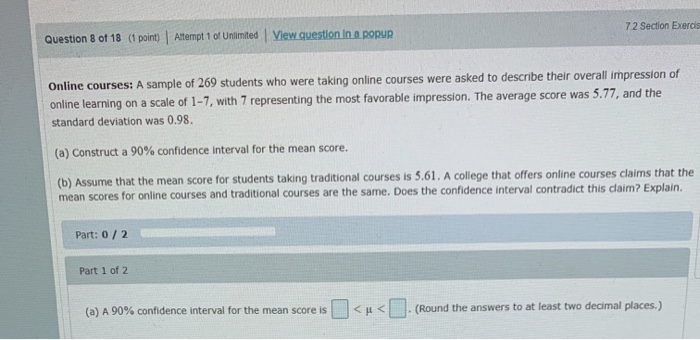Question 8 of 18 (1 point) Attempt 1 of Unlimited View question in a popur! 72 Section Exerci Online courses: A sample of 269 students who were taking online courses were asked to describe their overall impression of online learning on a scale of 1-7, with 7 representing the most favorable impression. The average score was 5.77, and the standard deviation was 0.98. (a) Construct a 90% confidence interval for the mean score. (b) Assume that the mean score for students taking traditional courses is 5.61. A college that offers online courses claims that the mean scores for online courses and traditional courses are the same. Does the confidence interval contradict this daim? Explain. Part: 0/2 Part 1 of 2 (a) A 90% confidence interval for the mean score is < < (Round the answers to at least two decimal places.)

a) At 90% confidence level, the critical value is z0.05 = 1.645

The 90% confidence interval is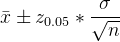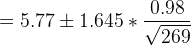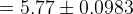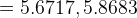b) Since the interval does not contain 5.61, so the confidence interval contradict the claim.

Earn Coins

Coins can be redeemed for fabulous gifts.

Similar Homework Help Questions
• Question 7 of 22 (1 point) Attempt 1 of Unlimited View question in a pour 82...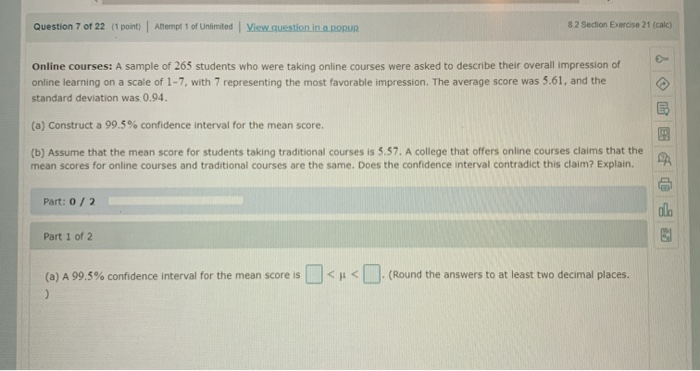Question 7 of 22 (1 point) Attempt 1 of Unlimited View question in a pour 82 Section Exercise 21 (cale) Online courses: A sample of 265 students who were taking online courses were asked to describe their overall impression of online learning on a scale of 1-7, with 7 representing the most favorable impression. The average score was 5.61, and the standard deviation was 0.94. (a) Construct a 99.5% confidence interval for the mean score. (b) Assume that the mean...

• A sample of 250 students who were taking online courses were asked to describe their overall...

A sample of 250 students who were taking online courses were asked to describe their overall impression of online learning on a scale of 1-7 with 7 representing the most favorable impression. The average score was 4.98 and the standard deviation was 0.87. Construct a 90% confidence interval for the mean score. Round your final answers to 3 decimal places. The 90% confidence interval for the mean score is: ( , )

• Question 4 of 18 (1 point) Attempt 2 of Unlimited View question. In a popuR! 7.1 Section Exercise 37-38 A sample...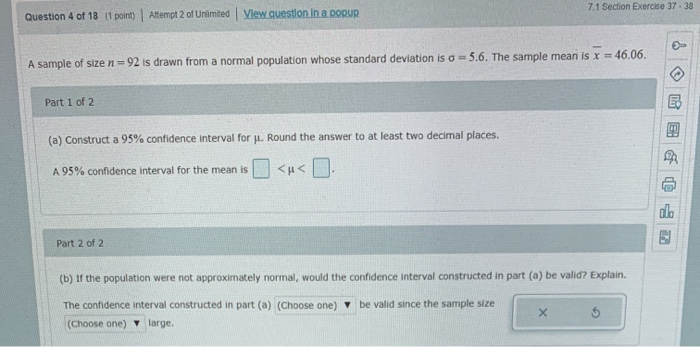Question 4 of 18 (1 point) Attempt 2 of Unlimited View question. In a popuR! 7.1 Section Exercise 37-38 A sample of size n = 92 is drawn from a normal population whose standard deviation is a = 5.6. The sample mean is x = 46.06. Part 1 of 2 (a) Construct a 95% confidence interval for Jl. Round the answer to at least two decimal places. A 95% confidence interval for the mean is <u< . Part 2 of...

• Question 12 of 18 (1 point) Attempt 1 of Unlimited View question in a popur! 7.3...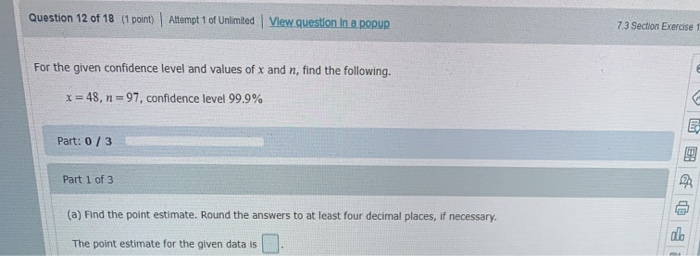Question 12 of 18 (1 point) Attempt 1 of Unlimited View question in a popur! 7.3 Section Exercise 1 For the given confidence level and values of x and n, find the following. x=48, n = 97, confidence level 99.9% Part: 0/3 Part 1 of 3 (a) Find the point estimate. Round the answers to at least four decimal places, if necessary. The point estimate for the given data is

• Question 1 of 13 (1 point) Attempt 1 of Unlimited View question in a popup 8.1...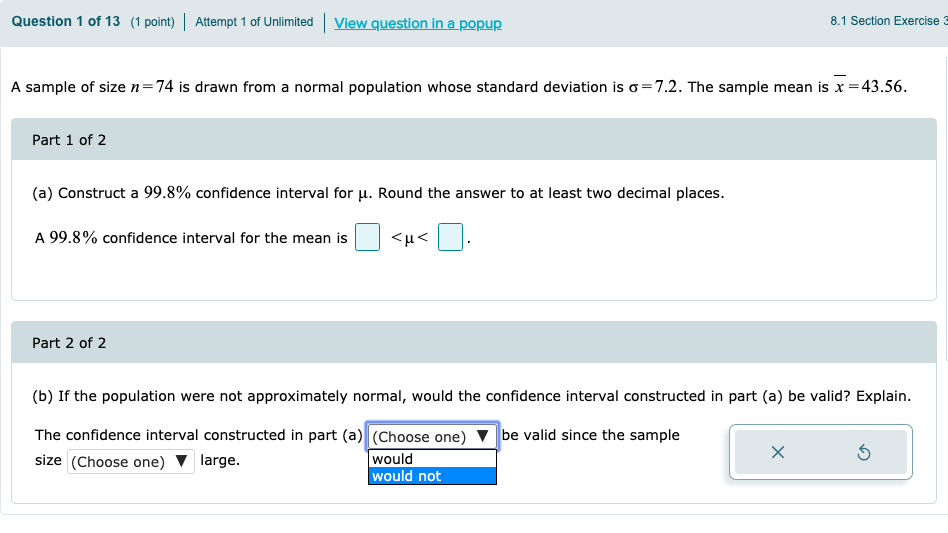Question 1 of 13 (1 point) Attempt 1 of Unlimited View question in a popup 8.1 Section Exercise A sample of size n=74 is drawn from a normal population whose standard deviation is o=7.2. The sample mean is x =43.56. Part 1 of 2 (a) Construct a 99.8% confidence interval for u. Round the answer to at least two decimal places. A 99.8% confidence interval for the mean is <u< . Part 2 of 2 (b) If the population were...

• Question 16 of 18 (1 point) Attempt 1 of Unlimited 74 Section Exercise 12 (cal Ages...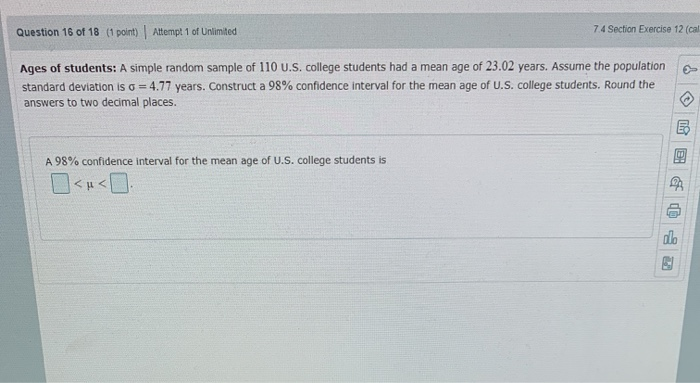Question 16 of 18 (1 point) Attempt 1 of Unlimited 74 Section Exercise 12 (cal Ages of students: A simple random sample of 110 U.S. college students had a mean age of 23.02 years. Assume the population standard deviation is o = 4.77 years. Construct a 98% confidence interval for the mean age of U.S. college students. Round the answers to two decimal places. A 98% confidence interval for the mean age of U.S. college students is

• 7.1 Section Exercise Question 3 of 18 (1 point) Altempt 3 of Unlimited View questlon in...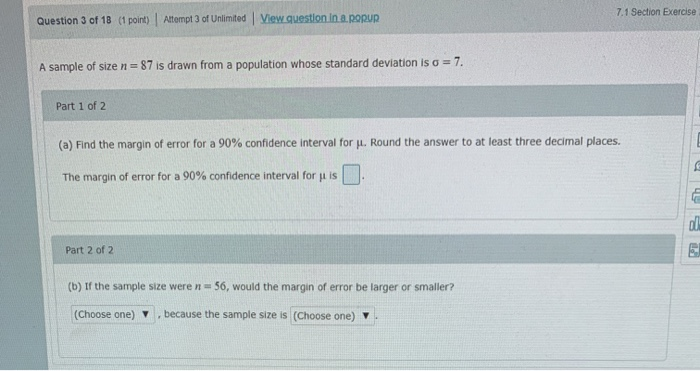7.1 Section Exercise Question 3 of 18 (1 point) Altempt 3 of Unlimited View questlon in a popup 7 A sample of size n 87 is drawn from a population whose standard deviation is a Part 1 of 2 (a) Find the margin of error for a 90% confidence interval for . Round the answer to at least three decimal places. The margin of error for a 90% confidence interval for u is Part 2 of 2 (b) If the...

• 0_U-IgNsikasNWEDBA9PVVRe4tCL6xedC30WT75R50Xmai_KAADILAjqzxPmXWKWIKC78EfixucBBnZ8UYHNdNiOwplSM. Homework 14 Question 8 of 11 (1 point) Question Attempt 2 of Unlimited ✓2 ✓3...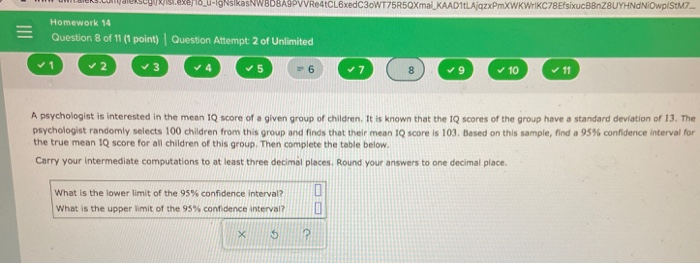0_U-IgNsikasNWEDBA9PVVRe4tCL6xedC30WT75R50Xmai_KAADILAjqzxPmXWKWIKC78EfixucBBnZ8UYHNdNiOwplSM. Homework 14 Question 8 of 11 (1 point) Question Attempt 2 of Unlimited ✓2 ✓3 ✓5 6 ✓7 8 ✓9 ✓ 10 ✓ 11 A psychologist is interested in the mean IQ score of a given group of children. It is known that the IQ scores of the group have a standard deviation of 13. The psychologist randomly selects 100 children from this group and finds that their mean IQ score is 103. Based on this sample, find a...

• Homework 12 Question 5 of 5 (1 point) Question Attempt 1 of Unlimited E The mean...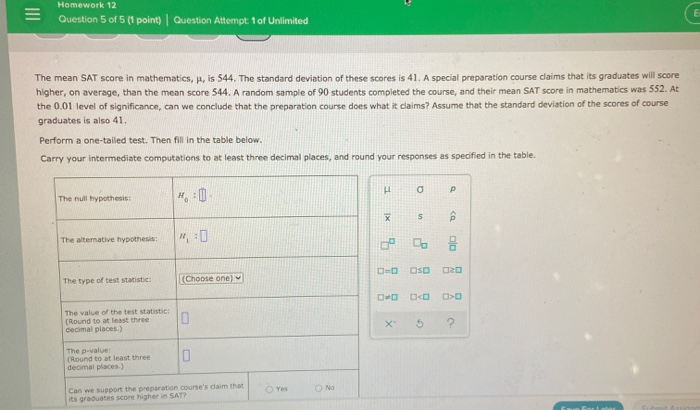Homework 12 Question 5 of 5 (1 point) Question Attempt 1 of Unlimited E The mean SAT score in mathematics, H, is 544. The standard deviation of these scores is 41. A special preparation course claims that its graduates will score higher, on average, than the mean score 544. A random sample of 90 students completed the course, and their mean SAT score in mathematics was 552. At the 0.01 level of significance, can we conclude that the preparation course...

• stion 14 of 18 (1 point) Attempt 1 of Unlimited View question in a popun iart...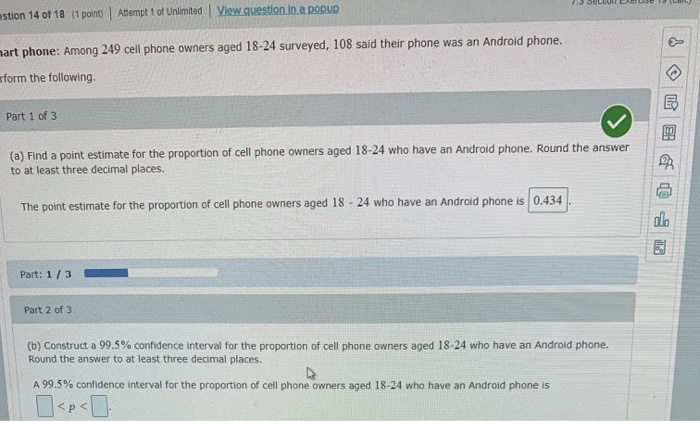stion 14 of 18 (1 point) Attempt 1 of Unlimited View question in a popun iart phone: Among 249 cell phone owners aged 18-24 surveyed, 108 said their phone was an Android phone. rform the following. Part 1 of 3 (a) Find a point estimate for the proportion of cell phone owners aged 18-24 who have an Android phone. Round the answer to at least three decimal places. The point estimate for the proportion of cell phone owners aged 18...